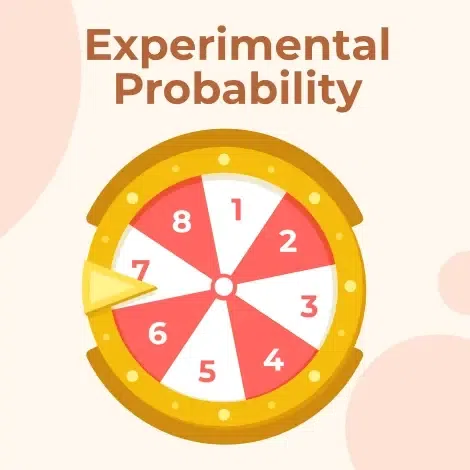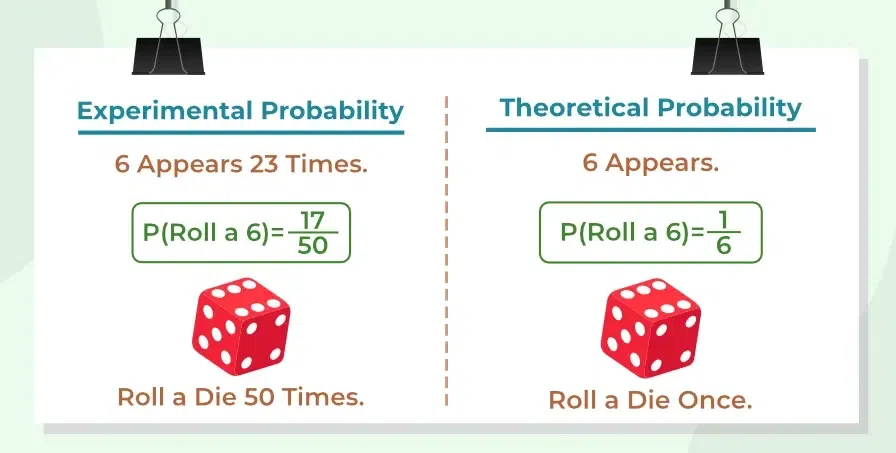GeeksforGeeks App
Open AppBrowser
Continue

# Experimental Probability

Probability means the chances of a number of occurrences of an event. In simple language, it is the possibility that an event will occur or not. The concept of probability can be applied to some experiments like coin tossing, dice throwing, playing cards, etc. Experimental Probability is one of the interesting concepts of Probability. Before diving down into the definition, Let’s start understanding this concept through our daily life situations.

We all have heard typical monsoon forecasts like, “Kerala remains under high alert expecting heavy rains and winds as a result of cyclone Burevi” and similar other headlines, right? But have you ever thought that how these expectations sometimes turn into reality? The reason behind the chances, expectations, doubts, and forecasts is Probability. Probability in simple meaning gives us the predictions of an event that may or may not be happened based on our past experiences. And these Past experience is based upon the experiment of events.

## What is Probability?

The branch of mathematics that tells us about the likelihood of the occurrence of any event is the probability. Probability tells us about the chances of happening an event. The probability of any element that is sure to occur is One(1) whereas the probability of any impossible event is Zero(0). The probability of all the elements ranges between 0 to 1.

There are two ways of studying probability that are

• Experimental Probability
• Theoretical Probability

Now let’s learn about both in detail.

## What is Experimental Probability?

Experimental probability is a type of probability that is calculated by conducting an actual experiment or by performing a series of trials to observe the occurrence of an event. It is also known as empirical probability.

To calculate experimental probability, you need to conduct an experiment by repeating the event multiple times and observing the outcomes. Then, you can find the probability of the event occurring by dividing the number of times the event occurred by the total number of trials.### Formula for Experimental Probability

The experimental Probability for Event A can be calculated as follows:

P(E) = (Number of times an event occur in an experiment) / (Total number of Trials)

### Examples of Experimental Probability

Now, as we learn the formula, let’s put this formula in our coin-tossing case.  If we tossed a coin 10 times and recorded a head 4 times and a tail 6 times then the Probability of Occurrence of Head on tossing a coin:

P(H) = 4/10

Similarly, the Probability of Occurrence of Tails on tossing a coin:

P(T) = 6/10

## What is Theoretical Probability?

Theoretical Probability deals with assumptions in order to avoid unfeasible or expensive repetition experiments. The theoretical Probability for an Event A can be calculated as follows:

P(A) = Number of outcomes favorable to Event A / Number of all possible outcomes

Now, as we learn the formula, let’s put this formula in our coin-tossing case. In tossing a coin, there are two outcomes: Head or Tail.

Hence, The Probability of occurrence of Head on tossing a coin is

P(H) = 1/2

Similarly, The Probability of the occurrence of a Tail on tossing a coin is

P(T) = 1/2## Experimental Probability vs Theoretical Probability

There are some key differences between Experimental and Theoretical Probability, some of which are as follows:

## Solved Examples of Experimental Probability

Example 1. Let’s take an example of tossing a coin, tossing it 40 times, and recording the observations. By using the formula, we can find the experimental probability for heads and tails as shown in the below table.

The formula for experimental probability:

P(H) = Number of Heads ÷ Total Number of Trials = 16 ÷ 40 = 0.4

Similarly,

P(H) = Number of Tails ÷ Total Number of Trials = 24 ÷ 40 = 0.6

P(H) + P(T) = 0.6 + 0.4 = 1

Note: Repeat this experiment for ‘n’ times and then you will find that the number of times increases, the fraction of experimental probability comes closer to 0.5. Thus if we add P(H) and P(T), we will get  0.6 + 0.4 = 1 which means P(H) and P(T) is the only possible outcomes.

Example 2. A manufacturer makes 50,000 cell phones every month. After inspecting 1000 phones, the manufacturer found that 30 phones are defective. What is the probability that you will buy a phone that is defective? Predict how many phones will be defective next month.

Experimental Probability = 30/1000 = 0.03

0.03 = (3/100) × 100 = 3%

The probability that you will buy a defective phone is 3%

⇒ Number of defective phones next month = 3% × 50000

⇒ Number of defective phones next month = 0.03 × 50000

⇒ Number of defective phones next month = 1500

Example 3. There are about 320 million people living in the USA. Pretend that a survey of 1 million people revealed that 300,000 people think that all cars should be electric. What is the probability that someone chosen randomly does not like the electric car? How many people like electric cars?

Now the number of people who do not like electric cars is 1000000 – 300000 = 700000

Experimental Probability =  700000/1000000 = 0.7

And, 0.7 = (7/10) × 100 = 70%

The probability that someone chose randomly does not like the electric car is 70%

The probability that someone like electric cars is  300000/1000000 = 0.3

Let x be the number of people who love electric cars

⇒ x = 0.3 × 320 million

⇒ x = 96 million

The number of people who love electric cars is 96 million.

## FAQs on Experimental Probability

### Q1: Define experimental probability.

Probability of an event based on an actual trail in physical world is called experimental probability.

### Q2: How is Experimental Probability calculated?

Experimental Probability is calculated using the following formula:

P(E) = (Number of trials taken in which event A happened) / Total number of trials

### Q3: Can Experimental Probability be used to predict future outcomes?

No,  experimental probability can’t be used to predict future outcomes as it only achives the theorectical value when the trails becomes infinity.

### Q4: How is Experimental Probability different from Theoretical Probability?

Theoretical probability is the probability of an event based on mathematical calculations and assumptions, whereas experimental probability is based on actual experiments or trials.

### Q5: What are some Limitations of Experimental Probability?

There are some limitation of experimental probability, which are as follows:

• Experimental probability can be influenced by various factors, such as the sample size, the selection process, and the conditions of the experiment.
• The number of trials conducted may not be sufficient to establish a reliable pattern, and the results may be subject to random variation.
• Experimental probability is also limited to the specific conditions of the experiment and may not be applicable in other contexts.

### Q6: Can Experimental Probability of an event be a negative number if not why?

As experimental probability is given by:

P(E) = Number of trials taken in which event A happened/Total number of trials

Thus, it can’t be negative as both number are count of something and counting numbers are 1, 2, 3, 4, …. and they are never negative.